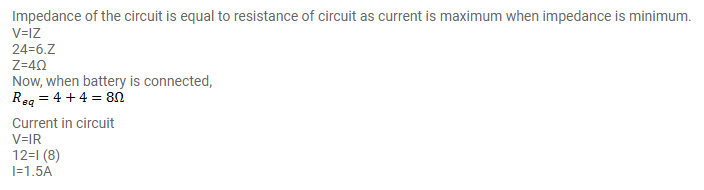# An inductor-coil, a capacitor and an AC source of rms voltage 24V

Question:

An inductor-coil, a capacitor and an AC source of rms voltage $24 \mathrm{~V}$ are connected in series. When the frequency of the source is varied, a maximum rms current of $6.0 \mathrm{~A}$ is observed. If this inductor coil is connected to a battery of emf $12 \mathrm{~V}$ and the internal resistance $4.0 \Omega$, what will be the current?

Solution: